# 3 digit by 3 digit Multiplication

### 3 digit by 3 digit Multiplication Examples

3 digit by 3 digit Multiplication – Example 1

Find the product.

235x 624

Explanation

Whenever we multiply a three digit Multiplicand by three-digit Multiplier, we first multiply the Multiplicand with one’s place of Multiplier, then with Tens place of the Multiplier and lastly by Hundreds place.

Step I: Multiply Multiplicand with one’s place of Multiplier.

i.e, 235 x 4 = 940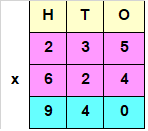Step II: Multiply Multiplicand with tens place of Multiplier.

i.e, 235 x 2 = 470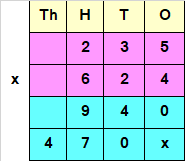Since the Number obtained at Step II, has a Tens value, we add a “0” or “X” at the end of the answer obtained by multiplying Multiplicand with Tens place of the Multiplier.

Step III: Multiply Multiplicand with hundreds place of Multiplier.

i.e, 235 x 6 = 1410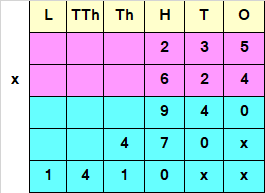Since the Number obtained at Step III, has a Hundreds place value, we add two “0” or “x” at the end of the answer obtained by multiplying Multiplicand with Tens place of the Multiplier.

Step III : Add all the numbers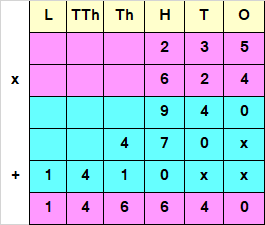Hence, 235 x 624 = 146640

3 digit by 3 digit Multiplication – Example 2

Find the product.

343 x 732

Explanation

Whenever we multiply a three digit Multiplicand by three digit Multiplier, we first multiply the Multiplicand with ones place of Multiplier, then with Tens place of the Multiplier and lastly by Hundreds place.

Step I : Multiply Multiplicand with ones place of Multiplier.

i.e, 343 x 2 = 686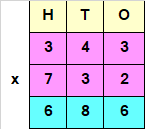Step II : Multiply Multiplicand with tens place of Multiplier.

i.e, 343 x 3 = 1029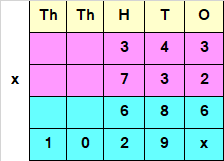Since the Number obtained at Step II, has a Tens value, we add a “0” or “X” at the end of the answer obtained by multiplying Multiplicand with Tens place of the Multiplier.

Step III : Multiply Multiplicand with hundreds place of Multiplier.

i.e, 343 x 7 = 2401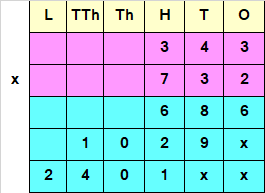Since the Number obtained at Step III, has a Hundreds place value, we add two “0” or “x” at the end of the answer obtained by multiplying Multiplicand with Tens place of the Multiplier.

Step III : Add all the numbers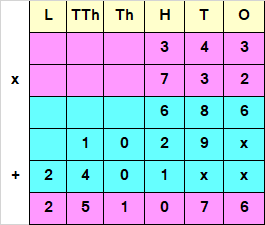Hence, 343 x 732 = 251076